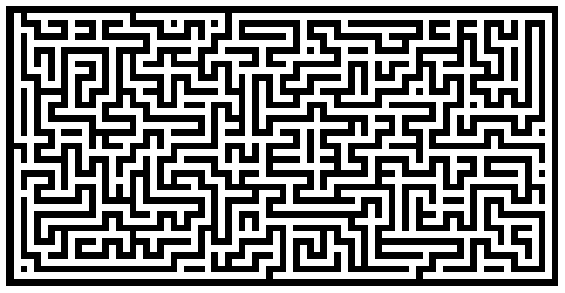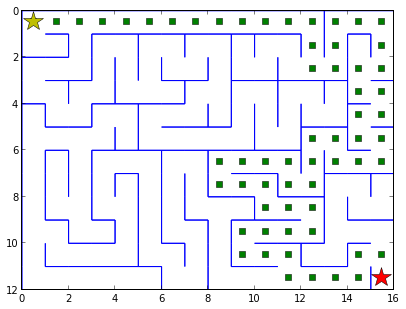In :
# from http://en.wikipedia.org/wiki/Maze_generation_algorithm
import numpy
from numpy.random import random_integers as rand
import matplotlib.pyplot as pyplot

def maze(width=81, height=51, complexity=.75, density=.75):
# Only odd shapes
shape = ((height // 2) * 2 + 1, (width // 2) * 2 + 1)
# Adjust complexity and density relative to maze size
complexity = int(complexity * (5 * (shape + shape)))
density    = int(density * (shape // 2 * shape // 2))
# Build actual maze
Z = numpy.zeros(shape, dtype=bool)
# Fill borders
Z[0, :] = Z[-1, :] = 1
Z[:, 0] = Z[:, -1] = 1
# Make aisles
for i in range(density):
x, y = rand(0, shape // 2) * 2, rand(0, shape // 2) * 2
Z[y, x] = 1
for j in range(complexity):
neighbours = []
if x > 1:             neighbours.append((y, x - 2))
if x < shape - 2:  neighbours.append((y, x + 2))
if y > 1:             neighbours.append((y - 2, x))
if y < shape - 2:  neighbours.append((y + 2, x))
if len(neighbours):
y_,x_ = neighbours[rand(0, len(neighbours) - 1)]
if Z[y_, x_] == 0:
Z[y_, x_] = 1
Z[y_ + (y - y_) // 2, x_ + (x - x_) // 2] = 1
x, y = x_, y_
return Z

pyplot.figure(figsize=(10, 5))
pyplot.imshow(maze(80, 40), cmap=pyplot.cm.binary, interpolation='nearest')
pyplot.xticks([]), pyplot.yticks([])
pyplot.show()In :
import numpy
from numpy.random import random_integers as rand
import matplotlib.pyplot as pyplot

class Cell:
def __init__(self, x, y):
self.x = x
self.y = y
self.backtrack = [0,0,0,0] #N,E,S,W
self.solution = [0,0,0,0]
self.walls = [1,1,1,1]
self.border = [0,0,0,0]
self.visited = False

def drawCell(self):
if self.walls == 1:
pyplot.hlines(y=self.y, xmin=self.x, xmax=self.x+1, linewidth=1, color='b')
if self.walls == 1:
pyplot.vlines(x=self.x+1, ymin=self.y, ymax=self.y+1, linewidth=1, color='b')
if self.walls == 1:
pyplot.hlines(y=self.y+1, xmin=self.x, xmax=self.x+1, linewidth=1, color='b')
if self.walls == 1:
pyplot.vlines(x=self.x, ymin=self.y, ymax=self.y+1, linewidth=1, color='b')

def setupBorder(self,xmax,ymax):
if self.y == 0: #N borders
self.border = 1
if self.x == xmax: #E borders
self.border = 1
if self.y == ymax: #S borders
self.border = 1
if self.x == 0: #W borders
self.border = 1

def maze(width=16, height=12):
# Build cells' fill
shape = (height, width)
Z = numpy.zeros(shape, dtype=bool)

# Initialize 2D cell arrray
cells = [[0 for y in xrange(0,height)] for x in xrange(0,width)]
# Initialize all walls (all present)
for y in xrange(0,height):
for x in xrange(0,width):
c = Cell(x,y)
c.setupBorder(width-1, height-1)
cells[x][y] = c

# Setup Cell Stack
stack = []
num_total = width*height
num_visited = 0 #keep track of total no. of cells visited
# Get random starting cell
rand_x = rand(0,width-1)
rand_y = rand(0,height-1)
#Z[rand_y,rand_x] = 1
print 'Starting cell:(',rand_x,',',rand_y,')'
current_cell = cells[rand_x][rand_y]
num_visited += 1

# DFS Algo
while num_visited < num_total:
neighbours_with_all_walls = checkNeighbours(cells, current_cell, current_cell.x, current_cell.y)
n = len(neighbours_with_all_walls)
if n > 0:
index = rand(0,n-1)
rand_neighbour = neighbours_with_all_walls[index]
# knock down the wall btw current cell and chosen neighbour
knockdownWallBtw(current_cell, rand_neighbour)
stack.append(current_cell)
current_cell = rand_neighbour
num_visited += 1
else:
cell = stack.pop()
current_cell = cell

# Final Drawing
for y in xrange(0,height):
for x in xrange(0,width):
c = cells[x][y]
c.drawCell()

# Route (given by end stack result)
#for c in stack:
#    pyplot.plot(c.x+0.5, c.y+0.5, linestyle='None', marker="s", color="y")

# Get Solution for given start/end point
calcSolution(cells, 0, 0, width-1, height-1)

return Z

def checkNeighbours(cells, current_cell, x, y):
neighbours = []
if current_cell.border == 0: #top
nt = cells[x][y-1]
if nt.walls == [1,1,1,1]:
neighbours.append(nt)
if current_cell.border == 0: #right
nr = cells[x+1][y]
if nr.walls == [1,1,1,1]:
neighbours.append(nr)
if current_cell.border == 0: #bottom
nb = cells[x][y+1]
if nb.walls == [1,1,1,1]:
neighbours.append(nb)
if current_cell.border == 0: #left
nl = cells[x-1][y]
if nl.walls == [1,1,1,1]:
neighbours.append(nl)
return neighbours

def knockdownWallBtw(cell, nbr):
#compare N,E,S,W direction to discern wall direction
if nbr.y == cell.y-1:
cell.walls = 0
nbr.walls = 0
return
if nbr.x == cell.x+1:
cell.walls = 0
nbr.walls = 0
return
if nbr.y == cell.y+1:
cell.walls = 0
nbr.walls = 0
return
if nbr.x == cell.x-1:
cell.walls = 0
nbr.walls = 0
return

# Gets the maze solution route for any given start/end point
def calcSolution(cells, startx, starty, endx, endy):
start_cell = cells[startx][starty]
end_cell = cells[endx][endy]

# DFS again...
stack = []
current_cell = start_cell
current_cell.visited = True
while current_cell != end_cell:
# get random possible neighbour
neighbours = getAllNeighboursNotYetVisited(cells, current_cell)
n = len(neighbours)
if n > 0:
index = rand(0,n-1)
rand_neighbour = neighbours[index]
rand_neighbour.visited = True
stack.append(current_cell)
current_cell = rand_neighbour
cell = stack.pop()
current_cell = cell

# Plot solution
for c in stack:
pyplot.plot(c.x+0.5, c.y+0.5, linestyle='None', marker="s", color="g")
pyplot.plot(start_cell.x+0.5, start_cell.y+0.5, linestyle='None', marker="*", color="y", markersize=20)
pyplot.plot(end_cell.x+0.5, end_cell.y+0.5, linestyle='None', marker="*", color="r", markersize=20)

def getAllNeighboursNotYetVisited(cells, c):
neighbours = []
if c.border == 0 and c.walls == 0:
n = cells[c.x][c.y-1]
if not n.visited:
neighbours.append(n)
if c.border == 0 and c.walls == 0:
n = cells[c.x+1][c.y]
if not n.visited:
neighbours.append(n)
if c.border == 0 and c.walls == 0:
n = cells[c.x][c.y+1]
if not n.visited:
neighbours.append(n)
if c.border == 0 and c.walls == 0:
n = cells[c.x-1][c.y]
if not n.visited:
neighbours.append(n)
return neighbours

pyplot.figure(figsize=(10, 5))
pyplot.imshow(maze(16, 12), cmap=pyplot.cm.binary, interpolation='nearest')
#pyplot.xticks([]), pyplot.yticks([])
pyplot.axis([0,16,12,0])
pyplot.show()

Starting cell:( 13 , 3 )In :